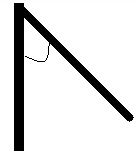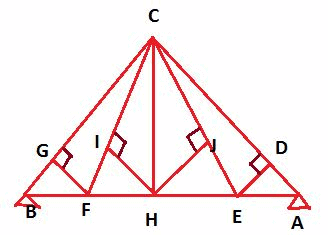Courses

# Test: Method Of Joints And Sections

## 17 Questions MCQ Test Structural Analysis I | Test: Method Of Joints And Sections

Description
This mock test of Test: Method Of Joints And Sections for Civil Engineering (CE) helps you for every Civil Engineering (CE) entrance exam. This contains 17 Multiple Choice Questions for Civil Engineering (CE) Test: Method Of Joints And Sections (mcq) to study with solutions a complete question bank. The solved questions answers in this Test: Method Of Joints And Sections quiz give you a good mix of easy questions and tough questions. Civil Engineering (CE) students definitely take this Test: Method Of Joints And Sections exercise for a better result in the exam. You can find other Test: Method Of Joints And Sections extra questions, long questions & short questions for Civil Engineering (CE) on EduRev as well by searching above.
QUESTION: 1

### How many equilibrium equations do we need to solve generally on each joint of a truss?

Solution:

Explanation: Summation of forces in x and y direction should be equated to 0. Since there is no bending moments in trusses, we don’t need to solve the third equation.

QUESTION: 2

### If a member of a truss is in compression, then what will be the direction of force that it will apply to the joints?

Solution:

Explanation: Member will apply outward force. Joint will in turn apply inward force resulting in compression of the member.

QUESTION: 3

### If a member of a truss is in tension, then what will be the direction of force that it will apply to the joints?

Solution:

Explanation: Member will apply inward force. Joint will in turn apply outward force resulting in compression of the member.

QUESTION: 4

What should be ideally the first step to approach to a problem using method of joints?

Solution:

Explanation: Identifying zero force members should always be the first step to approach any truss problem as it eliminate a lot of variables and is fairly easy.

QUESTION: 5

What should be the angle (in degrees) in the given system (part of a bigger system) if both of the members have to be a zero force member?Solution:

Explanation: 90o would mean that without any external force, each one would carry no force to satisfy equations of equilibrium.

QUESTION: 6In the above figure, force is applied at joint c and its magnitude is 10N with downward direction.

Q.  Which of the following are 0 force members?

Solution:

Explanation: FH, HE and AE are non-zero force member as there are directly transmitting load from the external support. So, by option elimination we can say that the answer is (a).

QUESTION: 7In the above figure, force is applied at joint c and its magnitude is 10N with downward direction.  .

Q.  What will the magnitude of force (in N) transmitted by FI?

Solution:

Explanation: GF is a zero force member as stated in earlier question. Now, in joint F, BF and FH are in a line. This means that the only remaining member FI which is not in line will transmit zero force.

QUESTION: 8In the above figure, force is applied at joint c and its magnitude is 10N with downward direction.  .

Q.  What will the magnitude of force (in N) transmitted by IC?

Solution:

Explanation: IH is a zero member force as is FI. So, IC too will be zero force members.

QUESTION: 9In the above figure, force is applied at joint c and its magnitude is 10N with downward direction.  .

Q.   What is total no. of zero force members in the above given system?

Solution:

Explanation: Following are the zero force member based on the logics explained above: – GF, HI, HJ, ED, FI, IC, CH, JE and JC.

QUESTION: 10

How many equilibrium equations are used in method of sections?

Solution:

Explanation: Moments too can be conserved along with forces in both directions. So, total no. of equations are three.

QUESTION: 11

In trusses, a member in the state of tension is subjected to:-

Solution:

Explanation: Pull is for tension, while push is for compression.

QUESTION: 12

In method of sections, what is the maximum no. of unknown members through which the imaginary section can pass?

Solution:

Explanation: Since we have three equilibrium equations, so we can have maximum 3 unknown forces/members through which imaginary section can pass.

QUESTION: 13

Method of substitute members is use for which type of trusses?

Solution:

Explanation: Method of substitute members is used to solve problems involving complex trusses.

QUESTION: 14

First step to solve complex truss using Method of substitute members is to convert it into unstable simple truss.
State whether the above statement is true or false.

Solution:

Explanation: First step is to convert it to stable simple truss.
Shear force is represented by V
Bending moment is represented by M
Distance along the truss is represented by X
W is the uniform load applied.

QUESTION: 15

On differentiating V wrt X we will get:-

Solution:

Explanation: On applying equilibrium equation, V – W(x)Δx – V – ΔV = 0.

QUESTION: 16

On differentiating M wrt X we will get:-

Solution: